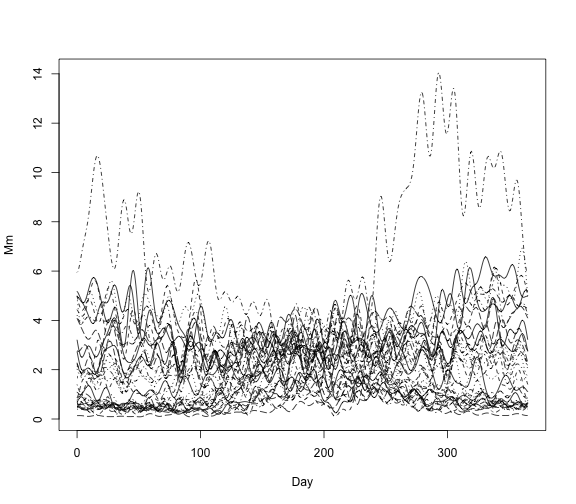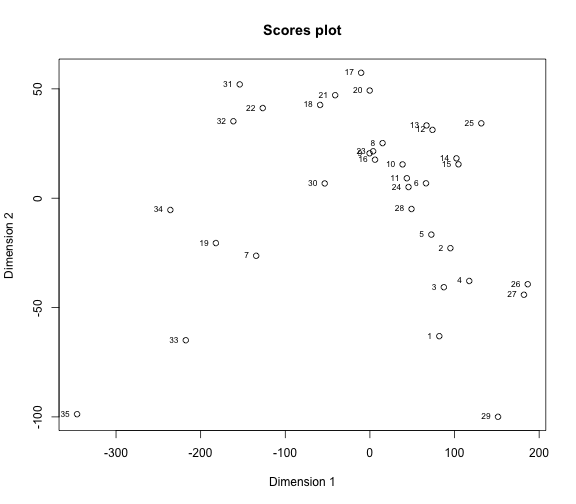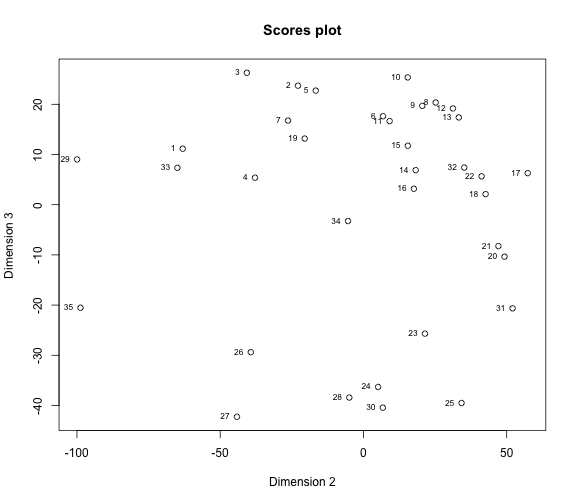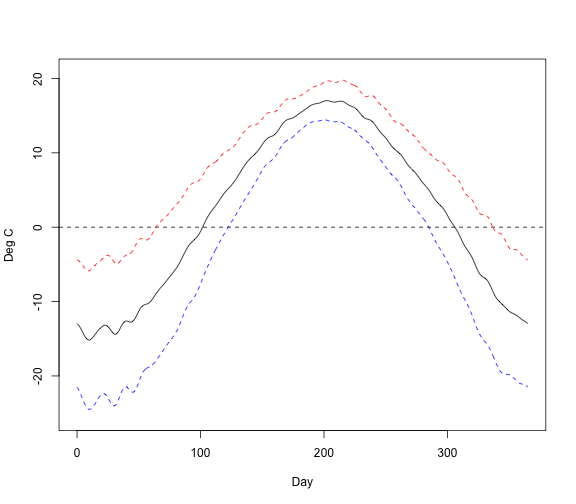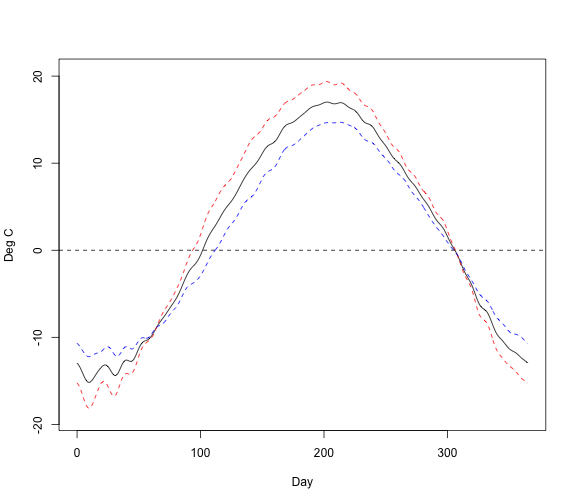# Description

The funHDDC algorithm (Schmutz et al., 2018) allows to cluster functional univariate or multivariate data by modeling each group within a specific functional subspace.

``````library(funHDDC)
``````

# Data

We are going to work on the Canadian Temperature data available in the fda package. It gathers temperature and pluviometry of 35 Canadian cities for one year.

``````library(fda)
#>
#> Attaching package: 'fda'
#> The following object is masked from 'package:graphics':
#>
#>     matplot
daybasis65 <- create.fourier.basis(c(0, 365), nbasis=65, period=365)
daytempfd <- smooth.basis(day.5, CanadianWeather\$dailyAv[,,"Temperature.C"], daybasis65,fdnames=list("Day", "Station", "Deg C"))\$fd
``````

# Example of Clustering univariate functional data

In this first part we are going to cluster data according to one functional variable: the temperature for one year.

## Basic code example

``````library(funHDDC)
#> Registered S3 method overwritten by 'funHDDC':
#>   method  from
#>   plot.fd fda
#>
#> Attaching package: 'funHDDC'
#> The following object is masked from 'package:fda':
#>
#>     plot.fd
res.uni<-funHDDC(daytempfd,K=3,model="AkBkQkDk",init="random",threshold=0.2)
#> funHDDC:
#>      model K threshold complexity        BIC
#> 1 AKBKQKDK 3       0.2        395 -12,720.19
#>
#> SELECTED: model  AKBKQKDK  with  3  clusters.
#> Selection Criterion: BIC.
``````

It prints the name of the model tested and the options chosen for the algorithm (K and the threshold for the scree test of Cattell), the complexity of the model chosen (i.e. the number of free model parameters) and the BIC value useful for model selection. It also prints the name of the best model according to the BIC criterion (in this example we test one model only so it is the same than the one tested).

Then you can plot the temperature curves colored by group.

``````plot(daytempfd,col=res.uni\$class)
``````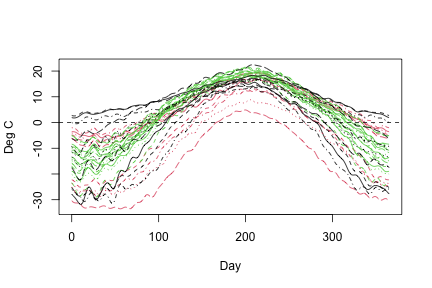``````#>  "done"
``````

## Selection of the number of clusters

You can use the BIC criterion in order to choose the best partition.

``````res.classif<-funHDDC(daytempfd,K=2:10,model="AkjBkQkDk")
#> funHDDC:
#>       model  K threshold complexity        BIC             comment
#> 1 AKJBKQKDK  7       0.2      1,114  -9,102.68
#> 2 AKJBKQKDK  5       0.2        915 -10,548.57
#> 3 AKJBKQKDK  6       0.2        982 -11,069.73
#> 4 AKJBKQKDK  3       0.2        523 -11,617.78
#> 5 AKJBKQKDK  2       0.2        263 -13,996.92
#> 6 AKJBKQKDK  4       0.2       <NA>       -Inf pop<min.individuals
#> 7 AKJBKQKDK  8       0.2       <NA>       -Inf pop<min.individuals
#> 8 AKJBKQKDK  9       0.2       <NA>       -Inf pop<min.individuals
#> 9 AKJBKQKDK 10       0.2       <NA>       -Inf pop<min.individuals
#>
#> SELECTED: model  AKJBKQKDK  with  7  clusters.
#> Selection Criterion: BIC.
``````

The model does not converge for all partitions. The model stored in res.classif is the best one according to the BIC criterion.

# Example of clustering bivariate functional data

In this part we are going to cluster data according to 2 functional variables: temperature and pluviometry in order to highlight different type of cities.

## Basic code example

The kmeans initialization allows to speed up convergence of the model.

``````res.multi<-funHDDC(list(daytempfd,dayprecfd),K=4,model="AkBkQkDk",init="kmeans",threshold=0.2)
#> Warning in funHDDC(list(daytempfd, dayprecfd), K = 4, model = "AkBkQkDk", : All
#> models diverged.
``````

Then you can plot the temperature curves and the precipitation curves colored by group.

``````plot(daytempfd,col=c("black","red","#009933","#FFCC00")[res.multi\$class],ylab="Temperature (Deg C)")
``````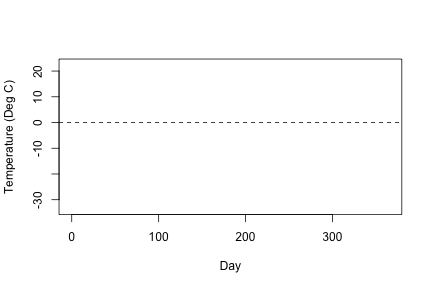``````#>  "done"
plot(dayprecfd,col=c("black","red","#009933","#FFCC00")[res.multi\$class],ylab="Precipitation (mm)")
``````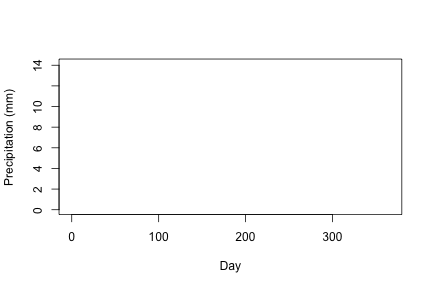``````#>  "done"
``````

## Selection of the number of clusters

As in the previous example you can select the best partition with the BIC criterion or you can use the slope heuristic criterion. A comparision of those two criteria is provided in Schmutz et al. (2018).

In this example we will test multiple number of clusters in order to see which partition is the best for data.

``````res.classif<-funHDDC(list(daytempfd,dayprecfd),K=2:8,model="AkBkQkDk",init="kmeans")
#> funHDDC:
#>      model K threshold complexity        BIC             comment
#> 1 AKBKQKDK 4       0.2      1,557 -20,346.51
#> 2 AKBKQKDK 6       0.2      1,954 -21,638.99
#> 3 AKBKQKDK 3       0.2      1,041 -22,747.90
#> 4 AKBKQKDK 2       0.2        523 -26,303.56
#> 5 AKBKQKDK 5       0.2       <NA>       -Inf pop<min.individuals
#> 6 AKBKQKDK 7       0.2       <NA>       -Inf pop<min.individuals
#> 7 AKBKQKDK 8       0.2       <NA>       -Inf       unknown error
#>
#> SELECTED: model  AKBKQKDK  with  4  clusters.
#> Selection Criterion: BIC.
``````

According to the BIC, the best model is the one with 4 clusters. With the slope heuristic :

``````slopeHeuristic(res.classif)
``````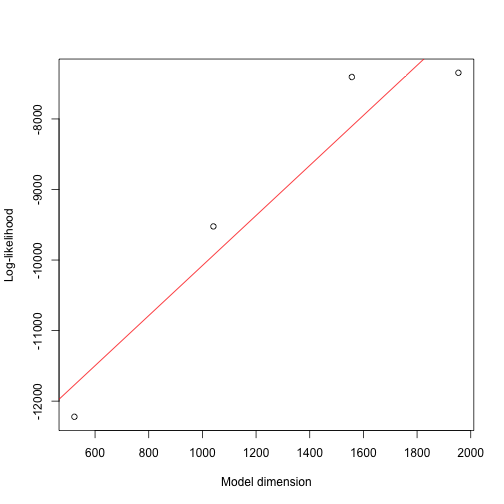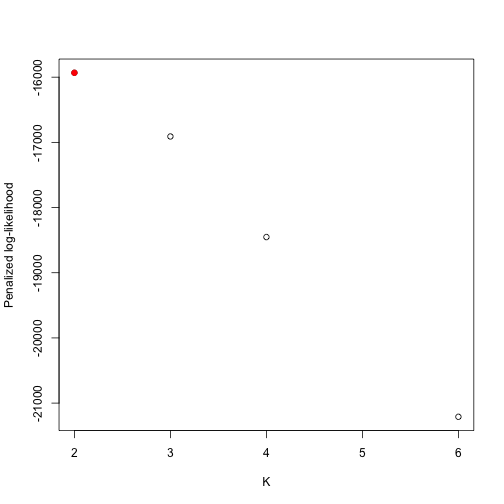``````#>  2
``````

The slopeHeuristic function provides 2 graphics, the first one show the maximum log-likelihood with regard to the free model parameters for each partition. The red line is estimated using a robust linear regression and its coefficient is used to compute the penalized log-likelihood function shown on the right plot. In this example the slope heuristic suggests 2 clusters. It is not the best criterion to use because there is not a great number of partitions to test, so the log-likelihood does not reach a plateau. It is this plateau that we want to estimate with the linear regression (see Bouveyron et al (2015) for an example of a perfect graph).

## Model selection

funHDDC proposes 6 differents models, more or less parcimonious. Refer to Schmutz et al.(2018) for the interpretation of each model. As in the number of groups selection you can also use the BIC or the slope heuristic criterion to select the best model for your data. In this case wa are going to test all models in order to select the best one for data. In order to do that, you need to list all models you want to test as shown below:

``````mult.model<-funHDDC(list(daytempfd,dayprecfd),K=4,model=c("AkjBkQkDk","AkjBQkDk","AkBkQkDk","AkBQkDk","ABkQkDk","ABQkDk"))
#> funHDDC:
#>       model K threshold complexity        BIC
#> 1  AKBKQKDK 4       0.2      1,557 -20,346.51
#> 2 AKJBKQKDK 4       0.2      1,561 -20,354.33
#> 3   ABKQKDK 4       0.2      1,554 -20,372.57
#> 4  AKJBQKDK 4       0.2      1,430 -24,721.73
#> 5    ABQKDK 4       0.2      1,169 -25,880.56
#> 6   AKBQKDK 4       0.2      1,044 -27,631.99
#>
#> SELECTED: model  AKBKQKDK  with  4  clusters.
#> Selection Criterion: BIC.
``````

The second to last line of the output indicates the best model according to the BIC. If you choose the slope heuristic, the number on the x axis correspond to the number of the model written on the left of the table provided in funHDDC output (above).

``````slopeHeuristic(mult.model)
``````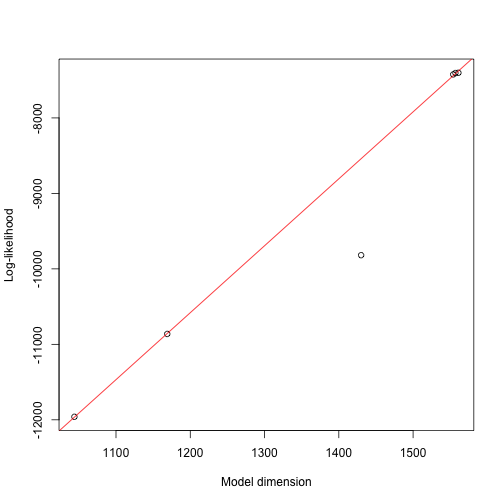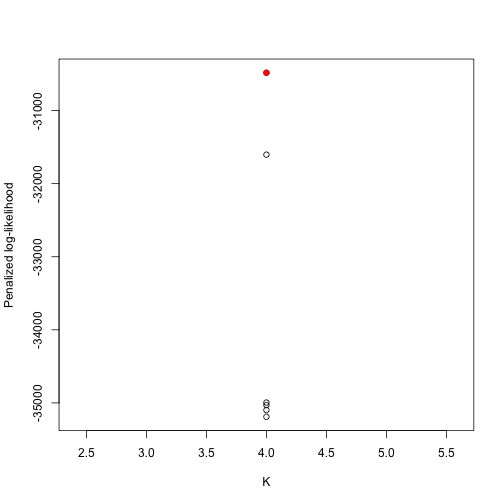``````#>  4
``````

##Multiple iterations of the funHDDC algorithm funHDDC function uses the EM algorithm for parameter estimation, this algorithm can reach sometimes some local maxima, that is why you may not find exactly the same result if you run the same code multiple times. So it is highly recommended to do multiple initialisations of the EM algorithm and choose the solution which maximizes the log-likelihood. By default, 20 initialisations of the EM algorithm are automatically done with the random initialization, and only the solution which maximizes the log-likelihood is displayed. If you want to increase the number of initialization or to do multiple initialisation for the kmeans initialisation, you have to use the nb.rep option.

``````multiple.init<-funHDDC(list(daytempfd,dayprecfd),K=4,init="kmeans",nb.rep=10)
``````

#Functional Principal Component Analysis Functional principal component analysis is one of the best known technique to do a first exploration of a functional dataset.

##Univariate case

``````res.pca<-mfpca(daytempfd)
plot.mfpca(res.pca)
``````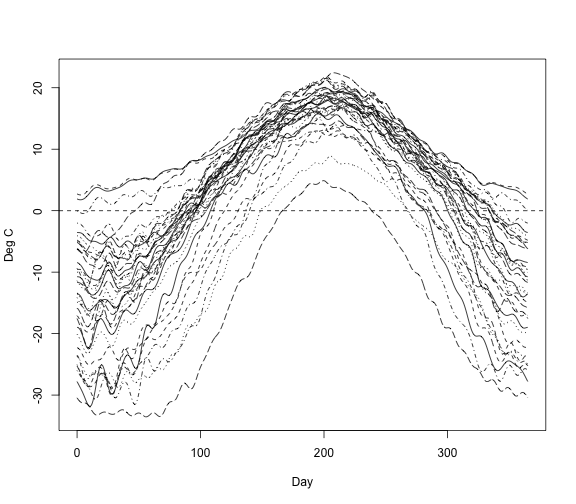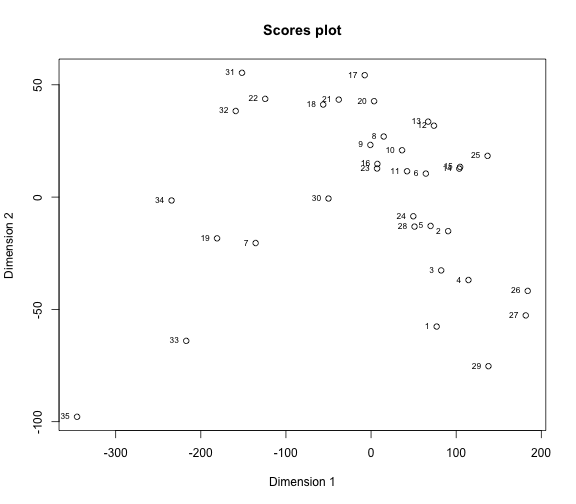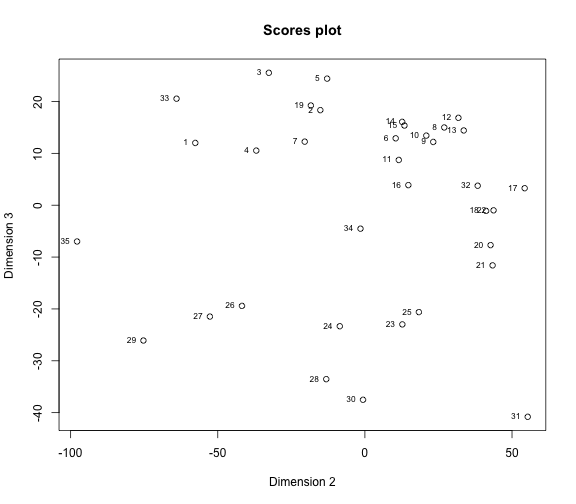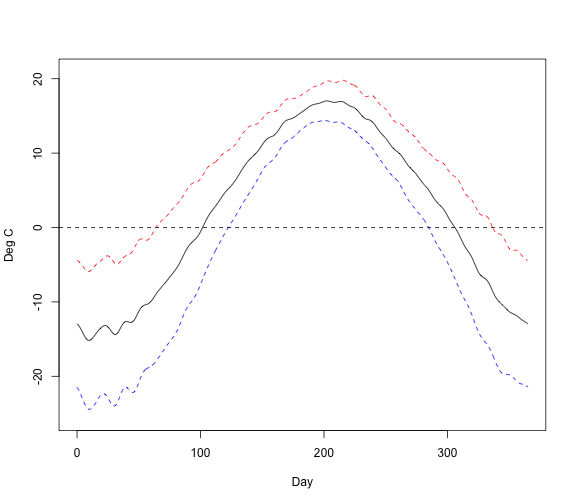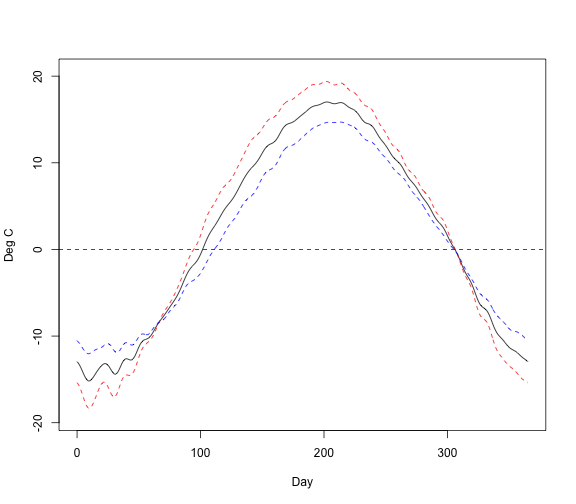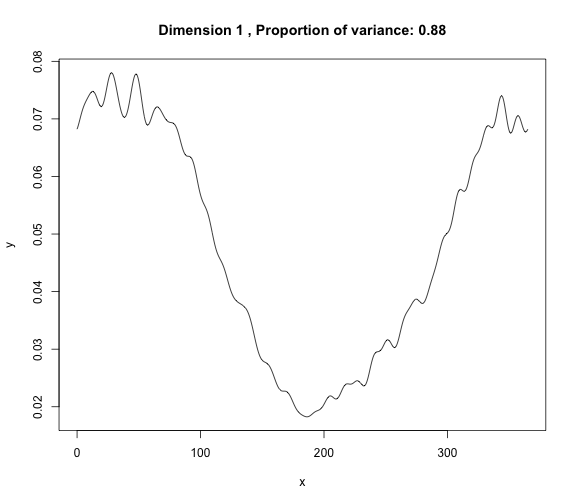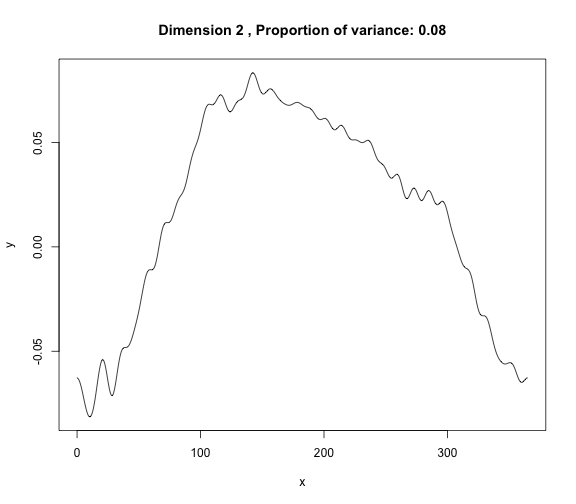The first plot corresponds to the smooth curves. The next plots are the scores projection on the 3 first dimensions (default value). Then there are the variation of mean curve (see Ramsay & Silvermann (2005) for a detailed interpretation). To end, there are the representation of the variation of the first two eigenfunctions.

##Multivariate case For the multivariate case, harmonics are build based on all functional variables. Then, except for scores plots, plots are displayed for each variable taken one by one.

``````res.pca<-mfpca(list(daytempfd,dayprecfd))
plot.mfpca(res.pca)
``````